NEET  >  Average Speed & Velocity and Instantaneous Speed & Velocity

# Average Speed & Velocity and Instantaneous Speed & Velocity Notes | Study Physics Class 11 - NEET

## Document Description: Average Speed & Velocity and Instantaneous Speed & Velocity for NEET 2022 is part of Physics Class 11 preparation. The notes and questions for Average Speed & Velocity and Instantaneous Speed & Velocity have been prepared according to the NEET exam syllabus. Information about Average Speed & Velocity and Instantaneous Speed & Velocity covers topics like Average Velocity and Average Speed & Velocity and Instantaneous Speed & Velocity Example, for NEET 2022 Exam. Find important definitions, questions, notes, meanings, examples, exercises and tests below for Average Speed & Velocity and Instantaneous Speed & Velocity.

Introduction of Average Speed & Velocity and Instantaneous Speed & Velocity in English is available as part of our Physics Class 11 for NEET & Average Speed & Velocity and Instantaneous Speed & Velocity in Hindi for Physics Class 11 course. Download more important topics related with notes, lectures and mock test series for NEET Exam by signing up for free. NEET: Average Speed & Velocity and Instantaneous Speed & Velocity Notes | Study Physics Class 11 - NEET
 1 Crore+ students have signed up on EduRev. Have you?

Suppose an object is in motion, the position of that object changes with time. But how fast is the position changing with time and in what direction? To understand this, we define average velocity and average speed.

Average Velocity

Average velocity is the ratio of total displacement to total time. Its direction is the same as the direction of the moving object. Even if the object is slowing down, and the magnitude of the velocity is decreasing, its direction would be still the same as the direction in which the object is moving. The magnitude of average velocity is always less than or equal to the average speed because displacement is always smaller than or equal to distance.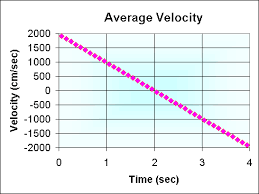It is a vector quantity and has units of m/s
Average velocity = Total Displacement / Total Time
The average velocity of the particle can be positive as well as negative and its positive and negative value depends on the sign of displacement. If the displacement of the particle is zero its average velocity is also zero.

Calculating Average Velocity

The velocity of a particle is: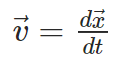Average Speed

The total distance travelled by the body in total time is the average speed.
Average speed = Total Displacement / Total Time
It is a scalar quantity and its units are m/s.

Calculating Average Speed

Average speed is found by the first finding the total distance covered by the object and dividing it by the total time taken in travelling the distance. Example: A body covers a circle of radius 100m on 100s, implies the total distance covered by him will be d = 2πr = 200π m. So, the average speed will be v = d/t = 2π m/s
If the motion of an object is along a straight line and in the same direction, the magnitude of displacement is equal to the total path length. In that case, the magnitude of the average velocity is equal to the average speed. In case the average speed is not equal to the magnitude of the average velocity, this is because the motion involves a change in direction and so path length is greater than the magnitude of displacement. So the average speed is greater than the magnitude of the velocity.

Solved Examples For You
Q. A particle moves for 20swith velocity 3m/s and then moves with velocity 4m/s for another 20s and finally moves with velocity 5m/s for next 20s what is the average velocity of the particle? (in m/s)
(a) 3
(b) 5
(c) 4
(d) zero
Ans:
c

Q.2 An insect crawling straight down the length of a meter stick is at the 12cm mark at one instant, and 22 minutes later is at the 60cm mark. Which one of the following is the magnitude of the insect’s average velocity?
(a) 0.4cm/s
(b) 0.5cm/s
(c) 24cm/s
(d) 30cm/s

Ans: A. The total displacement of insect is S = 60 - 12 = 48cm
Total time taken t = 2min = 120s
Insect’s average velocity = 48/120 = 0.4 cm/s

Instantaneous Speed and Velocity

Let’s carry out a small activity. You are walking towards theatre from home. Suddenly you realize that you are running late and would probably miss the beginning. You start running pretty fast. Maybe it’s been a while since the last time you ran and you slow down a bit. This is ‘instantaneous speed’.  And if you include the direction with that speed then you get the instantaneous velocity.

Instantaneous velocity

The rate of change of displacement of an object in a particular direction is its velocity. Its S.I unit is meter per second.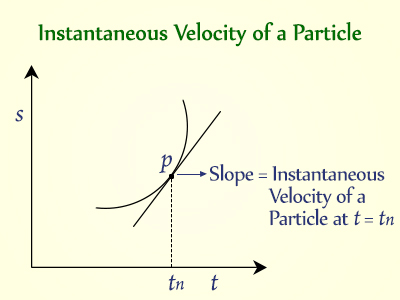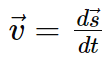The direction of instantaneous velocity at any time gives the direction of motion of a particle at that point in time. The magnitude of instantaneous velocity equals the instantaneous speed. This happens because, for an infinitesimally small time interval, the motion of a particle can be approximated to be uniform.

Instantaneous Speed

The average velocity tells us how fast an object has been moving over a given time interval but does not tell us how fast it moves at different instants of time during that interval. For this, we define instantaneous speed. It is the rate of change of distance with respect to time.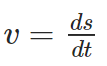Instantaneous speed is always greater than or equal to zero and is a scalar quantity. For uniform motion, instantaneous speed is constant. To understand it in simple words we can say that instantaneous speed at any given time is the magnitude of instantaneous velocity at that time. It is a limit of the average speed as the time interval become very small.
A moving object does not have the same speed during its travel. Sometimes it speeds up and sometimes slows down. At a given instant time what we read from the speedometer is instantaneous speed. When a cop pulls you over for speeding, he clocked your car’s instantaneous speed or speed at a specific point in time as your car sped down the road.

Solved Examples For You

Q. Which of the following sentences is an example of instantaneous velocity?
(i) The car covered 500 kilometers first 10 hours of its northward journey.
(ii) Five seconds into launch, the rocket was shooting upward at 5000 meters per second.
(iii) The Cheetah can run at 70 miles per hour.
(iv) Moving at 5 kilometres per hour, it will take us eight hours to get to the base.
(v) Roger Bannister was the first person to run one mile in less than four minutes.
Ans:
b

The document Average Speed & Velocity and Instantaneous Speed & Velocity Notes | Study Physics Class 11 - NEET is a part of the NEET Course Physics Class 11.
All you need of NEET at this link: NEET

## Physics Class 11

126 videos|449 docs|210 tests
 Use Code STAYHOME200 and get INR 200 additional OFF

## Physics Class 11

126 videos|449 docs|210 tests

### How to Prepare for NEET

Read our guide to prepare for NEET which is created by Toppers & the best Teachers

Track your progress, build streaks, highlight & save important lessons and more!

,

,

,

,

,

,

,

,

,

,

,

,

,

,

,

,

,

,

,

,

,

;